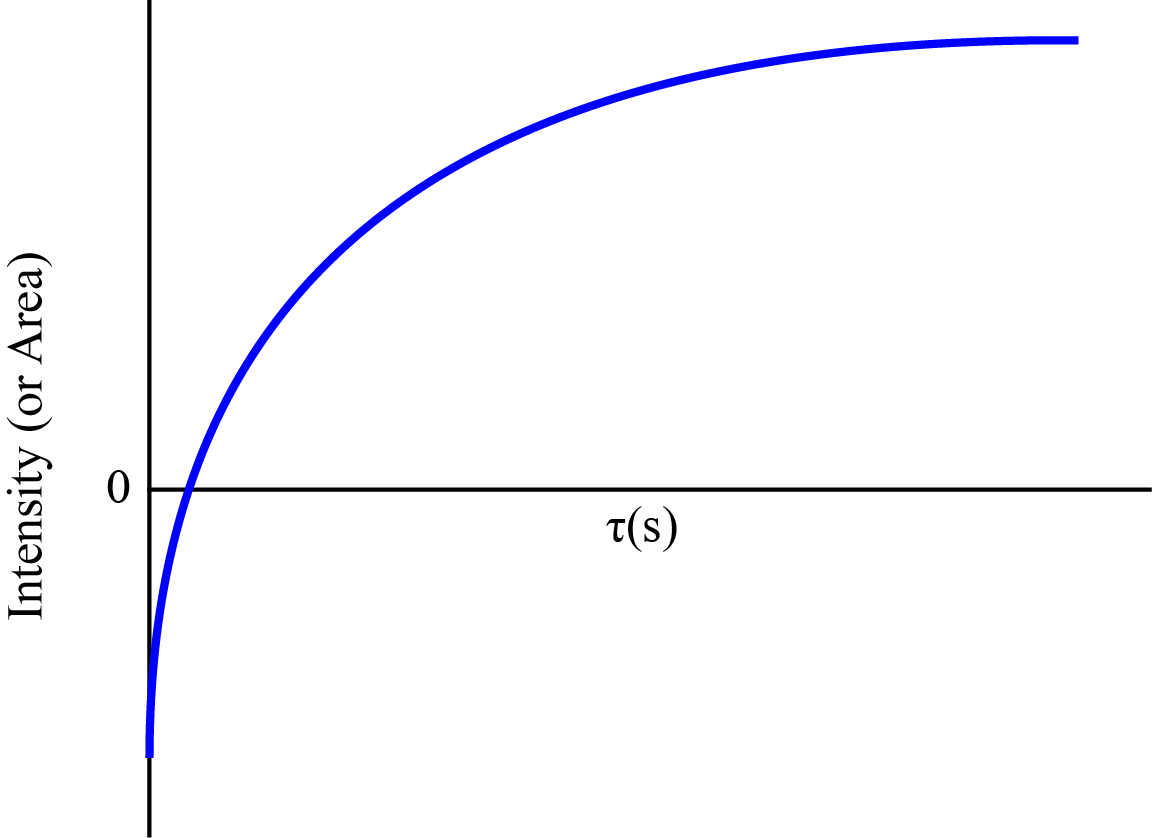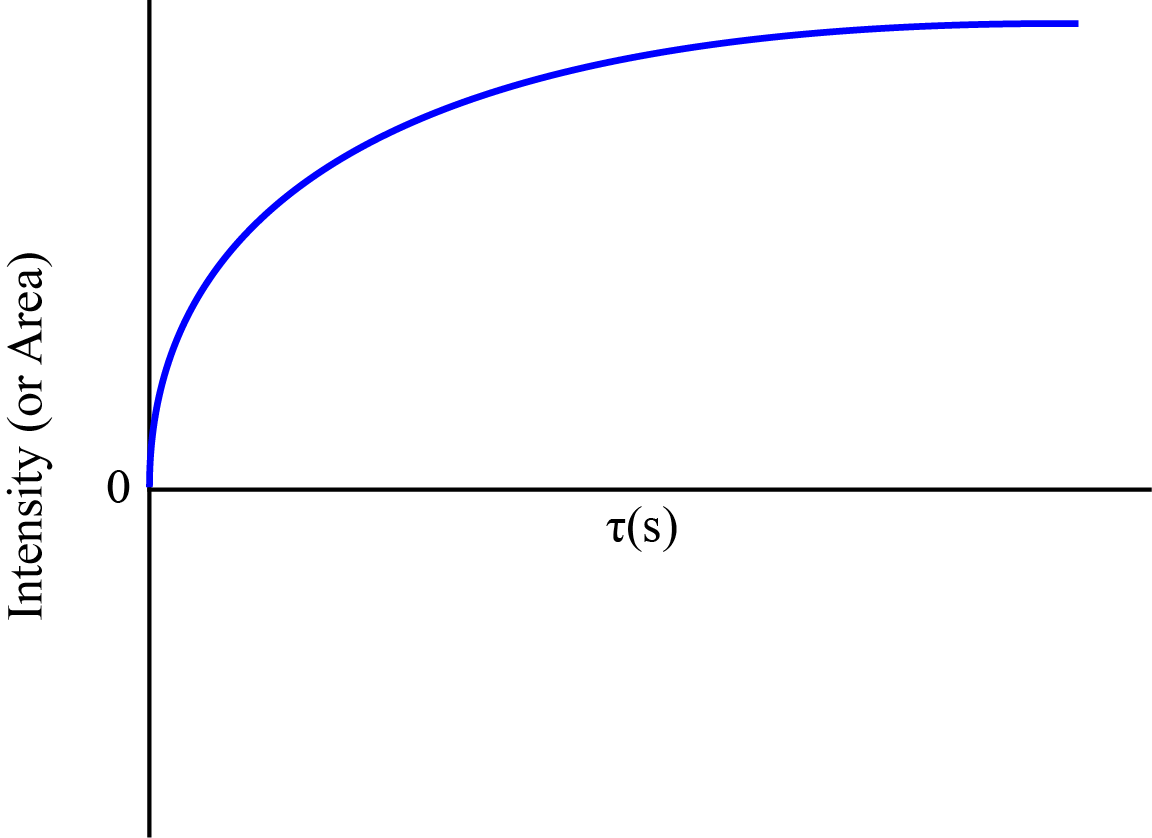Spin Lattice Relaxation

Page Under Construction!

Introduction

Spin lattice relaxation, more commonly referred to as T1is the time it takes for the bulk magnetization of the sample to recover 63% along the external magnetic field axis from the x-y plane. This section will be devoted to more intense aspects of spin-lattice relaxation, including relaxation mechanisms, relaxation theory, and how to measure T1 processes.

T1 Theory

Correlation Time

Discuss theory of what a correlation time is

Spectral Density

Explain the spectral density function

Temperature Effects

Explain effect of temperature

T1 Processes

There are many prcoesses that allow for spin lattice relaxation, paramgnetic impurities, coupling to the electric field gradient, dynamic motion, dipolar coupling, and others. Here I will attempt to outline the major T1 processes responsible for relaxation.

Spin 1/2

dipole-dipole, CSA, spin rotation

Spin>1/2

quadrupole, dipole-dipole, CSA, spin-rotation

T1 Measurements

There are two experiments commonly employed to measure the T1 for a compound, inversion recovery and saturation recovery. Each relies on having a rough idea of what the T1 should be.

Inversion Recovery

The inversion recovery experiment is an experiment to measure the T1 of a sample. The pulse sequence is shown belowFigure: Pulse sequence schematic showing the inversion recovery experiment. The gray dotted lines are $$\pi$$ /2 pulses that occur at different $$\tau$$ values.

The main idea behind the sequence is to invert the bulk magnetization using a $$\pi$$ pulse. Immediately after inversion, the spins being to recover along the z-axis. After a delay $$\tau$$, the spins are irradiated with a $$\pi/2$$ pulse. The delay is variable and produces the following spectra based on tau.Figure: A graphic depicting the how the bulk magnetization changes with varying delays and pulses. The signal is first inverted and once the delay between the pulses is sufficient, the magnetization becomes positive.

After the experiment is complete, plotting the intensities vs the $$\tau$$ delay will result in a curve that looks something likeFigure: A plot of the intensity vs the delay times of the experiment.

For most compounds, this line can be fit to an exponential function in the form of

$M_z(t)=M_0-2M_0e^{-t/T_1}$

where T1 is the spin lattice relaxation time.

Saturation Recovery

The saturation recovery experiment is another experiment which can be used to measure T1. This method is particularly useful if the lineshape is very broad, as is the case for quadupolar nuclei, or if the signal can't be inverted. The pulse sequence, represented below, consists of a train of (\frac{\pi}{2}\) pulses followed by a (\frac{\pi}{2}\) pulse after a delay $$\tau$$. The magnetization is knocked into the x-y plane and held there during the pulse comb. Then the magnetization recovers during the $$\tau$$ delay.Figure:FigureFigure

References

1. This is meant for references used for constructing the module. They must be primary and accessible to readers at a library.
2. You need at least two different sources here. Websites are not allowed. DOI links to J. Chem. Ed. are ideal Do not reference class notes. Also, do not reference textbooks for maximal credit. Using the insert citation button to automatically handle references is highly suggested (bottom right button on editor toolbar).